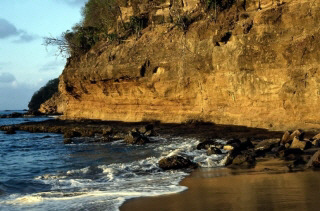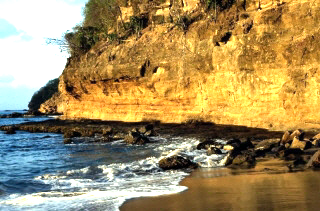﻿ ApplyMathematicalLogicCommand Class | Leadtools.ImageProcessing.Color | Raster, Medical, Document Help
←Select platform
In This Topic ▼

# ApplyMathematicalLogicCommand Class

Summary
Performs specific mathematical or logical operations on the colors of the image.
Syntax
C#
Objective-C
C++/CLI
Java
Python
``public class ApplyMathematicalLogicCommand : RasterCommand ``
``@interface LTApplyMathematicalLogicCommand : LTRasterCommand ``
````public class ApplyMathematicalLogicCommand `
`    extends RasterCommand ````
````public: `
`   ref class ApplyMathematicalLogicCommand : RasterCommand ````
``class ApplyMathematicalLogicCommand(RasterCommand): ``
Remarks
• This command supports 12 and 16-bit grayscale and 48 and 64-bit color images. Support for 12 and 16-bit grayscale and 48 and 64-bit color images is available only in the Document/Medical toolkits.
• This command does not support 32-bit grayscale images.

### Calculating Master Channel Values

In order to speed up widely used image processing filters in LEADTOOLS, the grayscale value (master channel) of a colored image is calculated using the following formulas:

````#define CalcGrayValue(r, g, b) ((L_UCHAR)(((L_UCHAR) (((2 * (L_UINT) (r)) + (5 * (L_UINT) (g)) + (L_UINT) (b) + 4) / 8)))) `
`#define CalcGrayValue16(r, g, b) ((L_UINT16) (((2 * (L_UINT32) (r)) + (5 * (L_UINT32) (g)) + (L_UINT32) (b) + 4) / 8)) `
`#define CalcGrayValue32(r, g, b) ((L_UINT32) (((2 * (L_UINT32) (r)) + (5 * (L_UINT32) (g)) + (L_UINT32) (b) + 4) / 8)) ````

Apply Mathematical Logic Function - BeforeApply Mathematical Logic Function - AfterView additional platform support for this Apply Mathematical Logic function.

Example

Run the ApplyMathematicalLogicCommand on an image.

C#
````using Leadtools; `
`using Leadtools.Codecs; `
`using Leadtools.ImageProcessing.Color; `
` `
` `
`public void ApplyMathematicalLogicCommandExample() `
`{ `
`   // Load an image `
`   RasterCodecs codecs = new RasterCodecs(); `
`   codecs.ThrowExceptionsOnInvalidImages = true; `
` `
`   RasterImage image = codecs.Load(Path.Combine(LEAD_VARS.ImagesDir, @"ImageProcessingDemo\NaturalFruits.jpg")); `
` `
`   // Prepare the command `
`   ApplyMathematicalLogicCommand command = new ApplyMathematicalLogicCommand(); `
`   command.Factor = 151; `
`   command.Flags = ApplyMathematicalLogicCommandFlags.OperationMultiply | ApplyMathematicalLogicCommandFlags.ValueDoNothing | ApplyMathematicalLogicCommandFlags.ResultDoNothing; `
`   // Multiply the colors of the bitmap using a factor of 1.51 . `
`   command.Run(image); `
`   codecs.Save(image, Path.Combine(LEAD_VARS.ImagesDir, "Result.jpg"), RasterImageFormat.Jpeg, 24); `
` `
`} `
` `
`static class LEAD_VARS `
`{ `
`   public const string ImagesDir = @"C:\LEADTOOLS22\Resources\Images"; `
`} ````
Requirements
Help Version 22.0.2023.3.31